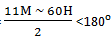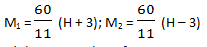# Concept & Tricks To Solve Clock Questions### Types of Questions

1. Position of hands of the clock or angle between them
2. Faulty clock

### Concept and Formula:

Minute hand traces in 60 minutes = hour hand traces in 12 hours = 360⁰
Angle of Hour space = 30⁰ Angle of minute space = 6⁰
Angle between minute hand and hour handExample:  angle between minute and hour hand at 10:27am
=In 12 hours = 11 times hands overlap
⇒ 180⁰angle between hands or in a straight line:
M =[Add if hour hand is at from 1 to 5; subtract if from 6 to 12]
In 12 hours = 11 times hands are in straight line

⇒ Both hands are perpendicular/ at 90⁰angle :
M =In 12 hours = 22 times hands are perpendicular to each other means in an hour, hands are perpendicular at two times so to find out exact timeNow, if value of (H ± 3) < 0 then adds 12 to find the exact value of minute
If value of (H ± 3) ≥ 12 then subtract 12 to find the exact value of minute

Example: To find the time between 9 to 10 o’clock when an hour and minute hands are perpendicular to each other.Between 9 to 10 o’clock at 9 o’clock and 9:32 o’clock both hands with be at a right angle.#### What's trending in BankExamsToday

Smart Prep Kit for Banking Exams by Ramandeep Singh - Download here